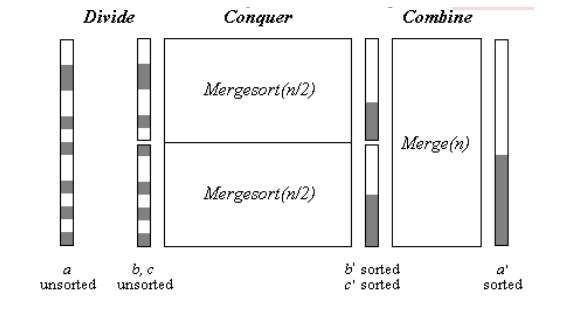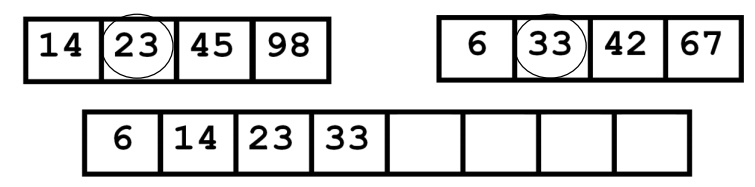# Merge sort

The Merge sort algorithm is based on divide and conquers strategy. First, the sequence to be sorted is decomposed into two halves ( Divide ). Each half is sorted independently ( Conquer ). Then the two sorted halves are merged to a sorted sequence ( Combine ).

MERGE SORT

Definition;

The Merge sort algorithm is based on divide and conquers strategy. First, the sequence to be sorted is decomposed into two halves ( Divide ). Each half is sorted independently ( Conquer ). Then the two sorted halves are merged to a sorted sequence ( Combine ).Algorithm:

The following procedure merge sort sorts a sequence a from index min to index max.

#include<stdio.h>

#include<conio.h>

void merge(int [],int ,int ,int );

void part(int [],int ,int );

int main()

{

int arr;

int i,size;

printf("\n\t------- Merge sorting method -------\n\n");

printf("Enter total no. of elements : ");

scanf("%d",&size);

for(i=0; i<size; i++)

{

printf("Enter %d element : ",i+1);

scanf("%d",&arr[i]);

}

part(arr,0,size-1);

printf("\n\t------- Merge sorted elements -------\n\n");

for(i=0; i<size; i++)

printf("%d ",arr[i]);

getch();

return 0;

}

void part(int arr[],int min,int max)

{

int mid;

if(min<max)

{

mid=(min+max)/2;

part(arr,min,mid);

part(arr,mid+1,max);

merge(arr,min,mid,max);

}

}

First, index m in the middle between lo and hi is determined. Then the first part of the sequence (from lo to m) and the second part (from m+1 to hi) are sorted by recursive calls of merge sort. Then the two sorted halves are merged by procedure merge. Recursion ends when lo = hi, i.e. when a subsequence consists of only one element.

The main work of the Merge sort algorithm is performed by function merge. There are different possibilities to implement this function.

The Merge method defined as follows,

void merge(int arr[],int min,int mid,int max)

{

int tmp;

int i,j,k,m;

j=min;

m=mid+1;

for(i=min; j<=mid && m<=max ; i++)

{

if(arr[j]<=arr[m])

{

tmp[i]=arr[j];

j++;

}

else

{

tmp[i]=arr[m];

m++;

}

}

if(j>mid)

{

for(k=m; k<=max; k++)

{

tmp[i]=arr[k];

i++;

}

}

else

{

for(k=j; k<=mid; k++)

{

tmp[i]=arr[k];

i++;

}

}

for(k=min; k<=max; k++)

arr[k]=tmp[k];

}

This variant of function merge requires in each case exactly the same number of steps –no matter if the input sequence is random, sorted or sorted in opposite direction.

Execution:Just like the above steps the second recursion will sort the sequence at the right hand side. Finally the both partitioned sorted sub arrays are get merged in the sorted sequence.

The final merging steps are as follows,14 and 6 are compared and 6 placed in the first position.

Next comparison will beNow comparing 14 and 33 14 is least value so place it in the second place. Next comparison,Likewise the comparisons done till reaches the end of the sub arrays and the list will be sorted at last, the sorted list will be as follows,Complexity Analysis:

The straightforward version of function merge requires at most 2n steps (n steps for copying the sequence to the intermediate array b, and at most n steps for copying it back to array a). The time complexity of merge sort is therefore

T(n) < = 2n + 2 T(n/2)   And

T(1)   = 0

The solution of this recursion yields

T(n)   < = 2n log(n)        include O(n log(n))

Thus, the merge sort algorithm is optimal, since the lower bound for the sorting problem of Ω (n log (n)) is attained.

In the more efficient variant, function merge requires at most 1.5n steps (n/2 steps for copying the first half of the sequence to the intermediate array b, n/2 steps for copying it back to array a, and at most n/2 steps for processing the second half). These yields a running time of merge sort of at most 1.5n log (n) steps.

Study Material, Lecturing Notes, Assignment, Reference, Wiki description explanation, brief detail
Programming and Data Structures : Sorting And Searching : Merge sort |